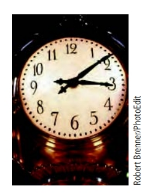Chapter 8.1, Problem 5ESMathematical Excursions (MindTap C...

4th Edition
Richard N. Aufmann + 3 others
ISBN: 9781305965584

Solutions

Chapter
SectionMathematical Excursions (MindTap C...

4th Edition
Richard N. Aufmann + 3 others
ISBN: 9781305965584
Textbook Problem

Evaluate each expression, where ⊕ and ⊖ indicate addition and subtraction, respectively, using a 12-hour clock.11 ⊕ 3

To determine

The addition 113 on a 12-hour clock.

Explanation

Given information: denote the addition on 12-hour.

Calculation:

113 denote the time 3 hours after 11 o’clock

Still sussing out bartleby?

Check out a sample textbook solution.

See a sample solution

The Solution to Your Study Problems

Bartleby provides explanations to thousands of textbook problems written by our experts, many with advanced degrees!

Get Started

In Exercises 19-24, find the functions f + g, f g, fg, and fg. 23. f(x) = x+1x1; g(x) = x+2x2

Applied Calculus for the Managerial, Life, and Social Sciences: A Brief Approach

A sample of n = 9 scores has X = 108. What is the sample mean?

Essentials of Statistics for The Behavioral Sciences (MindTap Course List)

Convert the expressions in Exercises 6584 to power form. xx

Finite Mathematics and Applied Calculus (MindTap Course List)